>>  <<  Usr  Pri  JfC  LJ  Phr  Dic  Voc  !:  Help  Learning J

# Chapter 3: Defining Functions

J comes with a collection of functions built-in; we have seen a few, such as * and +. In this section we take a first look at how to put together these built-in functions, in various ways, for the purpose of defining our own functions.

## 3.1 Renaming

The simplest way of defining a function is to give a name of our own choice to a built-in function. The definition is an assignment. For example, to define square to mean the same as the built-in *: function:
```   square =: *:

square 1 2 3 4
1 4 9 16
```
We might choose to do this if we prefer our own name as more memorable. We can give two different names to the same built-in function, intending to use one for the monadic case and the other for the dyadic.
```   Ceiling =: >.
Max     =: >.
```

 Ceiling 1.7 3 Max 4 2 4

## 3.2 Inserting

Recall that (+/ 2 3 4) means 2+3+4, and similarly (*/ 2 3 4) means 2*3*4. We can define a function and give it a name, say sum, with an assignment:
```   sum =: + /

sum 2 3 4
9
```
Here, sum =: +/ shows us that +/ is by itself an expression which denotes a function.

This expression +/ can be understood as: "Insert" (/) applied to the function + to produce a list-summing function.

That is, / is itself a kind of function. It takes one argument, on its left. Both its argument and its result are functions.

## 3.3 Terminology: Verbs, Operators and Adverbs

We have seen functions of two kinds. Firstly, there are "ordinary" functions, such as + and *, which compute numbers from numbers. In J these are called "verbs".

Secondly, we have functions, such as /, which compute functions from functions. Functions of this kind will be called "operators", to distinguish them from verbs.

Operators which take one argument are called "adverbs". An adverb always takes its argument on the left. Thus we say that in the expression (+ /) the adverb / is applied to the verb + to produce a list-summing verb.

The terminology comes from the grammar of English sentences: verbs act upon things and adverbs modify verbs.

## 3.4 Commuting

Having seen one adverb, (/), let us look at another. The adverb ~ has the effect of exchanging left and right arguments.

 'a' , 'b' 'a' ,~ 'b' ab ba

The scheme is that for a dyad f with arguments x and y

```             x f~ y      means    y f x
```
For another example, recall the residue verb | where 2 | 7 means, in conventional notation, "7 mod 2". We can define a mod verb:
```   mod =: | ~

```

 7 mod 2 2 | 7 1 1

Let me draw some pictures. Firstly, here is a diagram of function f applied to an argument y to produce a result (f y). In the diagram the function f is drawn as a rectangle and the arrows are arguments flowing into, or results flowing out of, the function. Each arrow is labelled with an expression.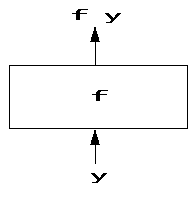Here is a similar diagram for a dyadic f applied to arguments x and y to produce (x f y).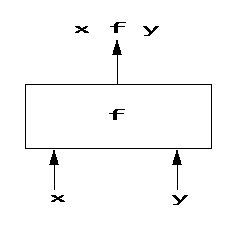Here now is a diagram for the function (f~), which can be pictured as containing inside itself the function f, together with a crossed arrangement of arrows.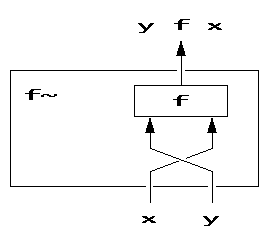## 3.5 Bonding

Suppose we wish to define a verb double such that double x means x * 2 . That is, double is to mean "multiply by 2". We define it like this:
```   double =: * & 2

double 3
6
```
Here we take a dyad, *, and produce from it a monad by fixing one of the two arguments at a chosen value (in this case, 2). The & operator is said to form a bond between a function and a value for one argument. The scheme is: if f is a dyadic function, and k is a value for the right argument of f, then
```            (f & k) y    means    y f k
```
Instead of fixing the right argument we could fix the left, so we also have the scheme
```            (k & f)  y   means    k f y
```
For example, suppose that the rate of sales tax is 10%, then a function to compute the tax, from the purchase-price is:
```   tax =: 0.10 & *

tax 50
5
```
Here is a diagram illustrating function k&f.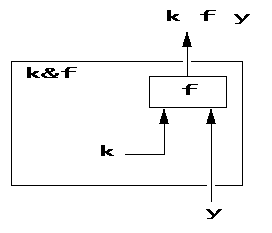## 3.6 Terminology: Conjunctions and Nouns

The expression (*&2) can be described by saying that the & operator is a function which is applied to two arguments (the verb * and the number 2), and the result is the "doubling" verb.

A two-argument operator such as & is called in J a "conjunction", because it conjoins its two arguments. By contrast, recall that adverbs are operators with only one argument.

Every function in J, whether built-in or user-defined, belongs to exactly one of the four classes: monadic verbs, dyadic verbs, adverbs or conjunctions. Here we regard an ambivalent symbol such as - as denoting two different verbs: monadic negation or dyadic subtraction.

Every expression in J has a value of some type. All values which are not functions are data (in fact, arrays, as we saw in the previous section).

In J, data values, that is, arrays, are called "nouns", in accordance with the English-grammar analogy. We can call something a noun to emphasize that it's not a verb, or an array to emphasize that it may have several dimensions.

## 3.7 Composition of Functions

Consider the English language expression: the sum of the squares of the numbers 1 2 3, that is, (1+4+9), or 14. Since we defined above verbs for sum and square, we are in a position to write the J expression as:
```   sum square 1 2 3
14
```
A single sum-of-the-squares function can be written as a composite of sum and square:
```   sumsq =: sum @: square

sumsq 1 2 3
14
```
The symbol @: (at colon) is called a "composition" operator. The scheme is that if f and g are verbs, then for any argument y
```           (f @: g) y    means  f (g y)
```
Here is a diagram for the scheme: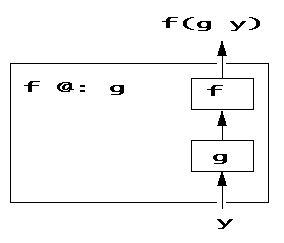At this point, the reader may be wondering why we write (f @: g) and not simply (f g) to denote composition. The short answer is that (f g) means something else, which we will come to.

For another example of composition, a temperature in degrees Fahrenheit can be converted to Celsius by composing together functions s to subtract 32 and m tomultiply by 5%9.

```   s       =: - & 32
m       =: * & (5%9)
convert =: m @: s

```

 s 212 m s 212 convert 212 180 100 100

For clarity, these examples showed composition of named functions. We can of course compose expressions denoting functions:

```   conv =: (* & (5%9)) @: (- & 32)
conv 212
100
```
We can apply an expression denoting a function, without giving it a name:
```   (* & (5%9)) @: (- & 32)  212
100
```
The examples above showed composing a monad with a monad. The next example shows we can compose a monad with a dyad. The general scheme is:
```                   x (f @: g) y   means    f (x g y)
```
For example, the total cost of an order for several items is given by multiplying quantities by corresponding unit prices, and then summing the results. To illustrate:
```   P =:  2 3        NB. prices
Q =:  1 100      NB. quantities

total =: sum @: *

```

 P Q P*Q sum P * Q P total Q 2 3 1 100 2 300 302 302

For more about composition, see Chapter 08.

## 3.8 Trains of Verbs

Consider the expression "no pain, no gain". This is a compressed idiomatic form, quite comprehensible even if not grammatical in construction - it is not a sentence, having no main verb. J has a similar notion: a compressed idiomatic form, based on a scheme for giving meaning to short lists of functions. We look at this next.

### 3.8.1 Hooks

Recall the verb tax we defined above to compute the amount of tax on a purchase, at a rate of 10%. The definition is repeated here:
```   tax =: 0.10 & *
```
The amount payable on a purchase is the purchase-price plus the computed tax. A verb to compute the amount payable can be written:
```   payable =: + tax
```
If the purchase price is, say, \$50, we see:

 tax 50 50 + tax 50 payable 50 5 55 55

In the definition (payable =: + tax) we have a sequence of two verbs + followed by tax. This sequence is isolated, by being on the right-hand side of the assignment. Such an isolated sequence of verbs is called a "train", and a train of 2 verbs is called a "hook".

We can also form a hook just by isolating the two verbs inside parentheses:

```   (+ tax) 50
55
```
The general scheme for a hook is that if f is a dyad and g is a monad, then for any argument y:
```            (f g) y       means   y f (g y)
```
Here is a diagram for the scheme: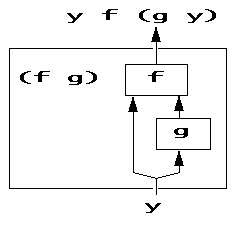For another example, recall that the "floor" verb <. computes the whole-number part of its argument. Then to test whether a number is a whole number or not, we can ask whether it is equal to its floor. A verb meaning "equal-to-its-floor" is the hook (= <.) :

```   wholenumber  =:  = <.

```

 y =: 3 2.7 <. y y = <. y wholenumber y 3 2.7 3 2 1 0 1 0

### 3.8.2 Forks

The arithmetic mean of a list of numbers L is given by the sum of L divided by the number of items in L. (Recall that number-of-items is given by the monadic verb #.)

 L =: 3 5 7 9 sum L # L (sum L) % (# L) 3 5 7 9 24 4 6

A verb to compute the mean as the sum divided by the number of items can be written as a sequence of three verbs: sum followed by % followed by # .

```   mean =: sum % #

mean L
6
```
An isolated sequence of three verbs is called a fork. The general scheme is that if f is a monad, g is a dyad and h is a monad then for any argument y,
```            (f g h) y     means   (f y) g (h y)
```
Here is a diagram of this scheme: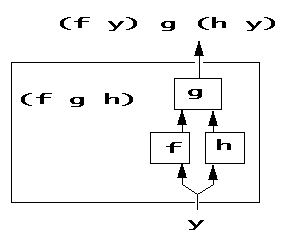For another example of a fork, what is called the range of numbers in a list is given by the fork smallest , largest where the middle verb is the comma.

Recall from Chapter 01 that the largest number in a list is given by the verb >./ and so the smallest will be given by <./

```   range =: <./  ,  >./

```

 L range L 3 5 7 9 3 9

Hooks and forks are sequences of verbs, also called "trains" of verbs. For more about trains, see Chapter 09.

## 3.9 Putting Things Together

Let us now try a longer example which puts together several of the ideas we saw above.

The idea is to define a verb to produce a simple display of a given list of numbers, showing for each number what it is as a percentage of the total.

Let me begin by showing you a complete program for this example, so you can see clearly where we are going. I don't expect you to study this in detail now, because explanation will be given below. Just note that we are looking at a program of 7 lines, defining a verb called display and its supporting functions.

```   frac     =: % +/
percent  =: (100 & *) @: frac
round    =: <. @: (+ & 0.5)
comp     =: round @: percent
br       =: ,.  ;  (,. @: comp)
tr       =: ('Data';'Percentages') & ,:
display  =: tr @: br
```
If we start with some very simple data:
```   data =: 3 1 4
```
then we see that the display verb shows each number as given and as a percentage (in round figures) of the total: 4 is 50% of 8.
```   display data
+----+-----------+
|Data|Percentages|
+----+-----------+
|3   |38         |
|1   |13         |
|4   |50         |
+----+-----------+
```
First, we aim to divide each number by the total, to show the contribution of each as a fraction. The hook (% +/) is suitable: it can be read as divide-by-sum. If we call it frac
```   frac =: % +/
```
then we see

 data +/data data % (+/data) frac data 3 1 4 8 0.375 0.125 0.5 0.375 0.125 0.5

The percentages are given by multiplying the fractions by 100.

```   percent  =: (100 & *) @: frac
```

 data frac data percent data 3 1 4 0.375 0.125 0.5 37.5 12.5 50

Let us round the percentages to the nearest whole number, by adding 0.5 to each and then taking the floor (the integer part) with the verb <. The verb round is:

```   round    =: <. @: (+&0.5)
```
Then the verb to compute the displayed values from the data is:
```   comp     =: round @: percent
```

 percent data round percent data comp data 37.5 12.5 50 38 13 50 38 13 50

Now we want to show the data and computed values in columns. To make a 1-column table out of a list, we can use the built-in verb ,. (comma dot, called "Ravel Items").

 data ,. data ,. comp data 3 1 4 3 1 4 38 13 50

To make the bottom row of the display, we define verb br as a fork which links together the data and the computed values, both as columns:

```   br  =: ,.  ;  (,. @: comp)
```

 data br data 3 1 4 +-+--+ |3|38| |1|13| |4|50| +-+--+

To add the top row of the display (the column headings), there is a useful built-in verb ,: (comma colon, "Laminate", which will be covered in Chapter 05)

```   tr  =: ('Data';'Percentages') & ,:

```

 data br data tr br data 3 1 4 +-+--+ |3|38| |1|13| |4|50| +-+--+ +----+-----------+ |Data|Percentages| +----+-----------+ |3   |38         | |1   |13         | |4   |50         | +----+-----------+

and so we put everything together:

```   display =: tr @: br

display data
+----+-----------+
|Data|Percentages|
+----+-----------+
|3   |38         |
|1   |13         |
|4   |50         |
+----+-----------+
```
This display verb has two aspects: the function comp which computes the values (the rounded percentages), and the remainder which is concerned to present the results. By changing the definition of comp, we can display a tabulation of the values of other functions. Suppose we define comp to be the built-in square-root verb (%:) .
```   comp =: %:
```
We would also want to change the column-headings in the top row, specified by the tr verb:
```   tr   =: ('Numbers';'Square Roots') & ,:

display 1 4 9 16
+-------+------------+
|Numbers|Square Roots|
+-------+------------+
| 1     |1           |
| 4     |2           |
| 9     |3           |
|16     |4           |
+-------+------------+
```
In review, we have seen a small J program with some characteristic features of J: bonding, composition, a hook and a fork. As with all J programs, this is only one of the many possible ways to write it.

In this chapter we have taken a first look at defining functions. There are two kinds of functions: verbs and operators. So far we have looked only at defining verbs. In the next chapter we look at another way of defining verbs, and in Chapter 13 onwards we will look at defining operators.

This is the end of Chapter 3.

The examples in this chapter were executed using J version 701. This chapter last updated 29 Jul 2012
Copyright © Roger Stokes 2012. This material may be freely reproduced, provided that this copyright notice is also reproduced.

>>  <<  Usr  Pri  JfC  LJ  Phr  Dic  Voc  !:  Help  Learning J